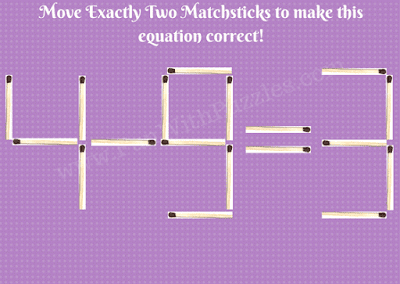Earlier we have published Matchstick Math puzzles for kids in which one has to move exactly one matchstick to make the equation. This was liked very much by the visitors of the Fun With Puzzles website. In the previously posted matchstick math puzzles, one has to move exactly one matchstick to make the equation correct. So we are extending this matchstick puzzle to move the two matches to make the equations correct. You can also check Matchstick Picture Puzzles in which your observational skills will be tested.
Here are a few Matchstick math equation puzzles. In each of these puzzles, one has to move exactly two matchsticks to different positives so that the equation given becomes correct. Some of these matchstick puzzles are easy. However, few of these puzzles are touch and will require out of box thinking.
Answers link to these puzzles is given at the end of this post. However, try to solve all of these puzzles without looking at answers and use the answers link to check the correctness of your answers. Also if you can find a different solution than what we have provided, please do post your comments about it.Matchstick Math Brain Teasers Picture-1Matchstick Math Brain Teasers Picture-2Matchstick Math Brain Teasers Picture-3Matchstick Math Brain Teasers Picture-4Matchstick Math Brain Teasers Picture-5

Do not forget to check out below mentioned Maths Puzzles and Brain Teasers to challenge your brain

## List of Maths Brain Teasers and Puzzles

Live to Learn said...

excellent

Heather said...

For the 1st Matchstick brain teaser, I believe you can change the first 5 into a 6 and then change the second 5 into a 9 to create 6 + 3 = 9.

Unknown said...

The second puzzle has alternative solutions 3 + 5 = 8 or 5 + 3 = 8 by just moving a single match to turn one of the 3’s into a 5.
But 2 matches must be moved, so create a dummy move. First move any other match elsewhere to turn one of the 3’s into a 9.
Second turn this 9 into a 5 by moving the match to location where the first match came from.

Rajesh Kumar said...

Great to know this alternative solution to the 2nd matchstick puzzle. This is your out-of-the-box thinking about completing two moves into the one move :).